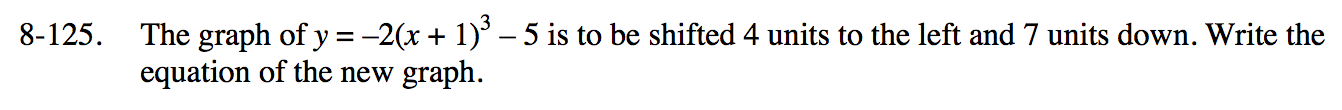### Home > PC3 > Chapter 8 > Lesson 8.3.3 > Problem8-125

8-125.

The graph of y = –2(x + 1)3 – 5 is to be shifted 4 units to the left and 7 units down. Write the equation of the new graph. Homework Help ✎Write your equation for the new graph. Then use a graphing calculator to graph the original equation and your shifted equation. Check your work, identifying and correcting any mistakes.